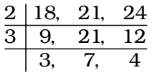## LCM and HCF

#### LCM and HCF

1. If P = 23.310.5 ; Q = 25.3.7, then HCF of P and Q is :

1. Given that , P = 23 × 310 × 5
Q = 25 × 3 × 7

##### Correct Option: B

Given that , P = 23 × 310 × 5
Q = 25 × 3 × 7
∴ HCF of P and Q = 23 × 3

1. The greatest number, that divides 122 and 243 leaving respectively 2 and 3 as remainders, is

1. As we know that the largest number which when divide the numbers a, b and c give remainders as p, q, r respectively is given by H.C.F. of (a – p), (b – q) and (c – r).
Clearly, 122 – 2 = 120 and 243 – 3 = 240 are exactly divisible by the required number.

##### Correct Option: D

As we know that the largest number which when divide the numbers a, b and c give remainders as p, q, r respectively is given by H.C.F. of (a – p), (b – q) and (c – r).
Clearly, 122 – 2 = 120 and 243 – 3 = 240 are exactly divisible by the required number.
∴ Required number = HCF of 120 and 240 = 120

1. Find the least multiple of 23, which when divided by 18, 21 and 24 leaves the remainder 7, 10 and 13 respectively.

1. We find LCM of 18, 21 and 24LCM of 18, 21 and 24 = 2 × 3 × 3 × 7 × 4 = 504
Now compare the divisors with their respective remainders. We observe that in all the cases the remainder is just 11 less than their respective divisor. So the number can be given by 504K – 11. Where K is a positive integer
Since 23 × 21 = 483
We can write 504K – 11 = (483 + 21)K – 11
⇒ 504K – 11 = 483 K + (21K – 11)
483 K is multiple of 23, since 483 is divisible by 23.
So, for (504K – 11) to be multiple of 23, the remainder (21K – 11) must be divisible by 23.

##### Correct Option: A

We find LCM of 18, 21 and 24LCM of 18, 21 and 24 = 2 × 3 × 3 × 7 × 4 = 504
Now compare the divisors with their respective remainders. We observe that in all the cases the remainder is just 11 less than their respective divisor. So the number can be given by 504K – 11. Where K is a positive integer
Since 23 × 21 = 483
We can write 504K – 11 = (483 + 21)K – 11
⇒ 504K – 11 = 483 K + (21K – 11)
483 K is multiple of 23, since 483 is divisible by 23.
So, for (504K – 11) to be multiple of 23, the remainder (21K – 11) must be divisible by 23.
Put the value of K = 1, 2, 3, 4, 5, 6, ..... and so on successively. We find that the minimum value of K for which (21K – 11) is divisible by 23. is 6, (21 × 6 – 11)
= 115 which is divisible by 23.
Therefore, the required least number = 504 × 6 – 11 = 3013

1. The least number to be subtracted from 36798 to get a number which is exactly divisible by 78 is

1. When 36798 is divided by 78, remainder = 60

##### Correct Option: B

When 36798 is divided by 78, remainder = 60
∴ The least number to be subtracted = 60

1. The LCM of two multiples of 12 is 1056. If one of the number is 132, the other number is

1. Let the numbers be 12p and 12q.
∴ Their LCM = 12pq , when p and q are prime to each other.

 ∴ q = 1056 = 8 [∴ 12p = 132 ] 132

##### Correct Option: C

Let the numbers be 12p and 12q.
∴ Their LCM = 12pq , when p and q are prime to each other.

 ∴ q = 1056 = 8 [∴ 12p = 132 ] 132

∴ Other number = 12q = 12 × 8 = 96